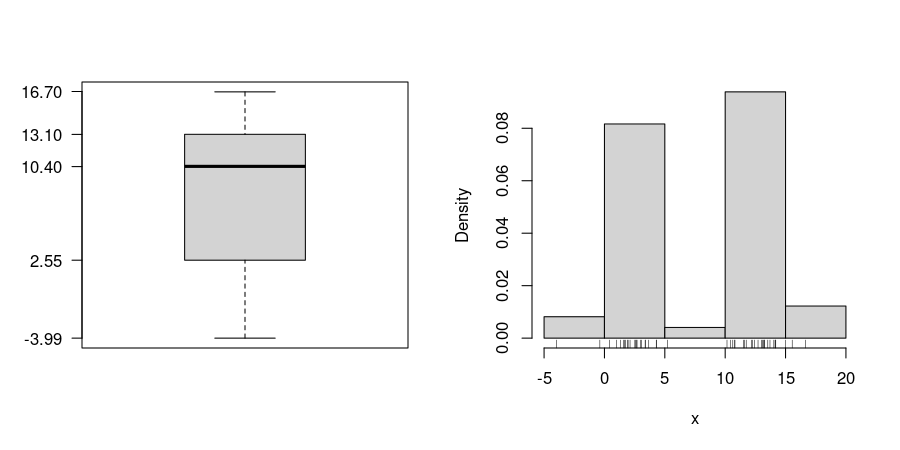## Exam 1

1. #### Question

For the 49 observations of the variable x in the data file boxhist.csv draw a histogram, a boxplot and a stripchart. Based on the graphics, answer the following questions or check the correct statements, respectively. (Comment: The tolerance for numeric answers is $±0.8$, the true/false statements are either about correct or clearly wrong.)
1. The distribution is unimodal. / The distribution is not unimodal.
2. The distribution is symmetric. / The distribution is right-skewed. / The distribution is left-skewed.
3. The boxplot shows outliers. / The boxplot shows no outliers.
4. A quarter of the observations is smaller than which value?
5. A quarter of the observations is greater than which value?
6. Half of the observations are smaller than which value?

#### Solution1. False / True
2. True / False / False
3. False / True
4. 2.55
5. 13.1
6. 10.4